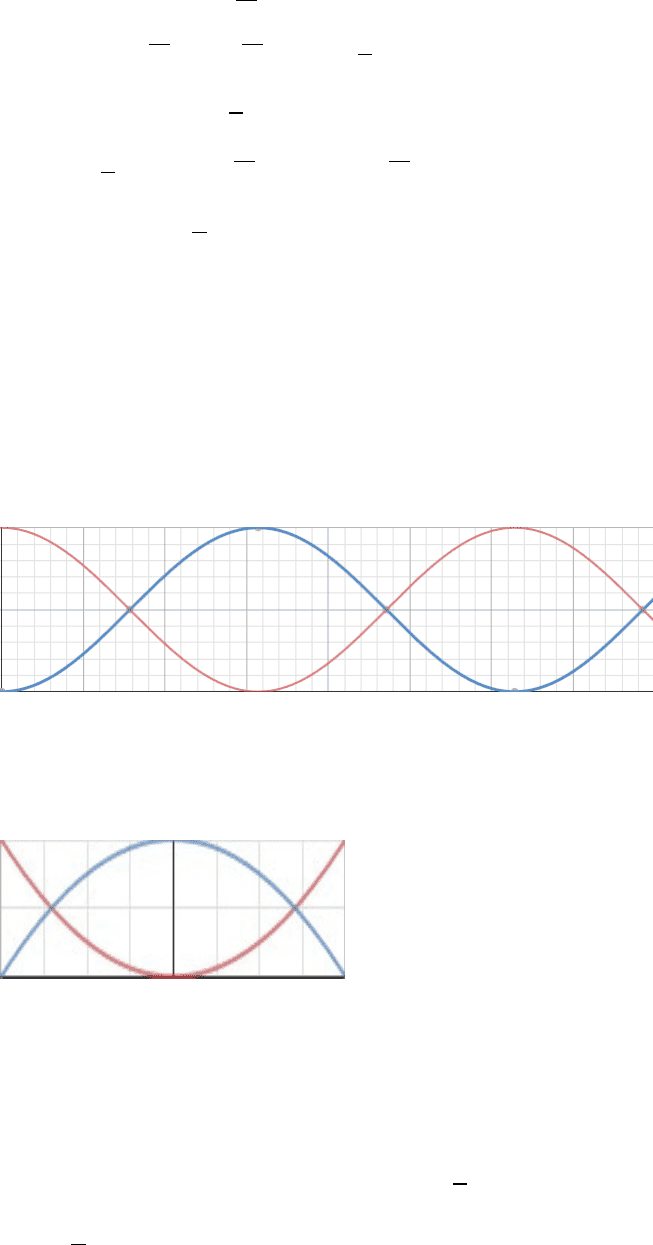# PHYS 101 Lecture Notes - Lecture 29: Angular Frequency, Propagation Constant, Simple Harmonic Motion

56 views5 pages

For unlimited access to Class Notes, a Class+ subscription is required.PHYS 101: Physics for the Life Sciences Lecture 29:
Simple Harmonic Motion
x(t)= Acos(ωt+δ)
V(t)=A ω sin(ωt +δ)
A(t)=A ω2sin (ωt +δ)
Acos(ωt +δ)¿2
PE=1
2k x2=1
2k¿
A ωsin (ωt +δ)¿2
KE=1
2mV 2=1
2m¿
Example:
Consider the following system (no friction)
Take as the system the spring and the mass
There is no friction, therefore friction does no work on the system.
The weight and normal force are perpendicular to the direction of displacement
and therefore they also do not work on the system.
Since there are no external forces acting on the system the Mechanical Energy is
conserved.
Emechanical=KE+PE+Ethermal
(
Ethermal =0
)
Acos (ω t +δ)¿2
A ωsin (ω t +δ)¿2+1
2k¿
¿1
2m¿
(Recall:
)
Unlock document

This preview shows pages 1-2 of the document.
Unlock all 5 pages and 3 million more documents.

Already have an account? Log inAcos((
)t+δ)¿2
A(
)sin((
)t+δ)¿2+1
2k¿
¿1
2m¿
¿1
2k A2[si n2(
t+δ)+co s2(
t+δ)]
(Recall:
si n2θ+co s2θ=1
)
Emechanical=1
2k A2
Graphic Representation of KE and PE:
Plot of KE and PE vs time (at
t=0
the spring is fully stretched)
Red Line = PE
Blue Line = KE
We can also plot KE and PE vs x
Red Line = PE
Blue Line = KE
Aside:
PE=¿
the potential energy in the spring
¿1
2k x2
KE=1
2mV 2
(we need to write this in terms of x)
Unlock document

This preview shows pages 1-2 of the document.
Unlock all 5 pages and 3 million more documents.

Already have an account? Log in

# Get access

Grade+
\$10 USD/m
Billed \$120 USD annually
Homework Help
Class Notes
Textbook Notes
40 Verified Answers
Study Guides
1 Booster Class
Class+
\$8 USD/m
Billed \$96 USD annually
Homework Help
Class Notes
Textbook Notes
30 Verified Answers
Study Guides
1 Booster Class